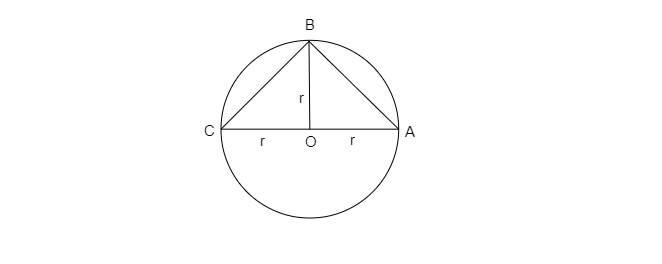# Find the area of the largest triangle that can be inscribed in a semi-circle of radius $r$ units, in square units.

Given: The largest triangle that can be inscribed in a semi-circle of radius $r$ units.

To do: To find the area of the triangle.

Solution:The area of a triangle is equal to the base times the height. In a semi circle, the diameter is the base of the semi-circle.

This is equal to $2\times r\ ( r=the\ radius)$

If the triangle is an isosceles triangle with an angle of $45^o$ at each end, then the height of the triangle is also a radius of the circle.

The base of the triangle is equal to $2\times r$, the height of the triangle is equal to $r$

Area of the triangle, $A=\frac{1}{2}\times b\times h$

$\Rightarrow A=\frac{1}{2}\times 2\times r\times r$

$\Rightarrow A=r^2$

Thus, the area of the largest triangle that can be inscribed in a semi-circle of radius $r$ units is $2r^2$ sq. units.